Homework 7: Class Groups and Elliptic Curves DUE WEDNESDAY, NOVEMBER 14

William Stein

Date: Math 124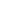HARVARD UNIVERSITYFall 2001

1.
(10 points) For any negative discriminant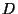, let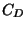denote the finite abelian group of equivalence classes of primitive positive definite quadratic forms of discriminant. Use the PARI program forms.gp from lecture 24 (download it from my web page) to compute representatives forand determine the structure ofas a produce of cyclic groups for each of the following five values of: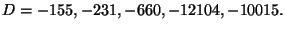2.
(6 points) Draw a beautiful graph of the set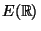of real points on each of the following elliptic curves:
(i)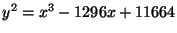,
(ii)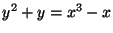,
(iii)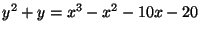.

3.
(4 points) A rational solution to the equation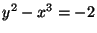is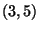. Find a rational solution with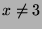by drawing the tangent line toand computing the third point of intersection.

William A Stein 2001-11-11